Reach Us+44-1474-556909
A Further Research on the Convergence of Wu-Schabacks Multi-quadric Quasi-Interpolation | OMICS International
Journal of Applied & Computational Mathematics
All submissions of the EM system will be redirected to Online Manuscript Submission System. Authors are requested to submit articles directly to Online Manuscript Submission System of respective journal.

# A Further Research on the Convergence of Wu-Schabacks Multi-quadric Quasi-Interpolation

Yang Zhang, Xue-Zhang Liang, Qiang Li*

School of Mathematics, Jilin University, Changchun, 130012, P.R. China

*Corresponding Author:
Qiang Li
School of Mathematics, Jilin University
Changchun, 130012, PR China
E-mail: [email protected]

Received August 25, 2013; Accepted September 23, 2013; Published September 26, 2013

Citation: Zhang Y, Zhang Y, Liang XZ, Li Q (2013) A Further Research on the Convergence of Wu-Schaback’s Multi-quadric Quasi-Interpolation J Appl Computat Math 2: 138. doi: 10.4172/2168-9679.1000138

Copyright: © 2013 Zhang Y, et al. This is an open-access article distributed under the terms of the Creative Commons Attribution License, which permits unrestricted use, distribution, and reproduction in any medium, provided the original author and source are credited.

Visit for more related articles at Journal of Applied & Computational Mathematics

#### Abstract

The paper discusses the error estimate of Wu-Schaback's quasi-interpolant for a wider class of approximated functions (the functions with lower smoothness order). Three cases are considered: a function with a Lipschitz continuous first-order derivative, a continuous function and a Lipschitz continuous function, respectively.

#### Keywords

Convergence; Wu-Schaback’s

#### Introduction

Quasi-interpolation methods have been used widely in data analysis, and have great values not only in theory but also in many application areas such as medicine, geology, economy and computer science. Multiquadric functions were first proposed by Hardy  in 1968, and Franke  showed they performed well in many calculations including the numerical experiments. Powell , Beatson and Powell , and Beatson and Dyn  successively proposed a number of quasiinterpolation schemes and discussed the convergence of the schemes. In 1994, Wu and Schaback  proposed a useful quasi-interpolation operator LDf and discussed the convergence and shape preserving properties of this operator. In their convergence theorem (theorem A in our paper), they claimed interpolated functions f(x)∈C2 . Based on these papers, Zhang and Wu , and Ma and Wu  did further researches. In this paper, we discuss the convergence of operator LDf for a wider range of approximated functions (namely functions with lower smoothness). To prove the convergence, we use two theorems showed by Beatson and Powell , and our method differs from that in . We assume that there are finite scattered points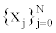in the bounded interval [a,b] as follows: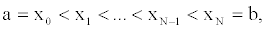and the maximum spacing is defined as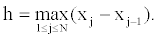For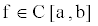, we define its norm as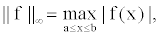and its modulus of continuity as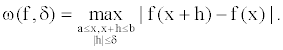The basis functions used in this paper are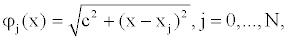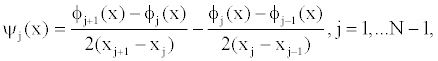where c>0 is a positive shape parameter

In 1994, Wu and Schaback proposed the quasi-interpolation operator LD: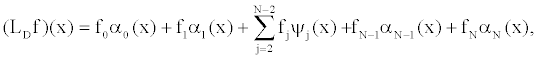where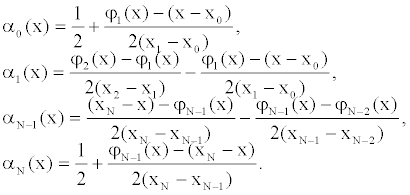They got the error estimate of this operator as follows:

Theorem A: For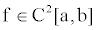the quasi-interpolant LD f satisfies an error estimate of type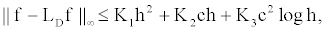where positive constants K1,K2,K3 are independent of h and c.

In 1992, Beatson and Powell  proposed the quasi-interpolation operator LB: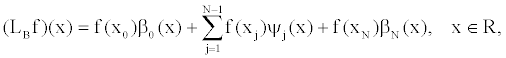where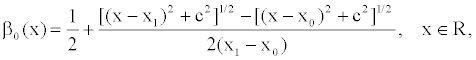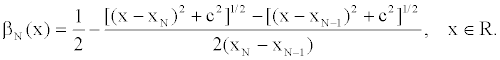They proved the following result:

Theorem B: In interval [a,b], the error function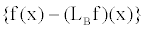satisfies the bound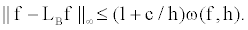Meanwhile, in  the quasi-interpolation operator LC was defined as follows: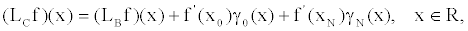where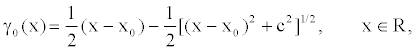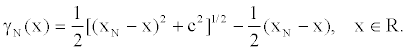They got the following theorem:

Theorem C: If f has a Lipschitz continuous first-order derivative, then the maximum error of the quasi-interpolant LCf satisfies the bound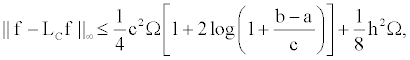where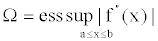It should be noticed that in Theorem A, Wu and Schaback demanded the approximated function f∈C2[a,b] . In this paper, we weaken this condition step by step. Using Theorem B and Theorem C proposed by Beatson and Powell, we get three theorems about convergence estimate for the approximated functions with lower smoothness.

Theorem 1: If f has a Lipschitz continuous first-order derivative, then we can draw the conclusion: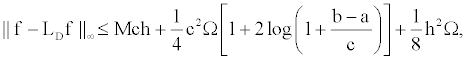where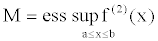Proof: We notice that quasi-interpolant LD f and LB f have the following relationship: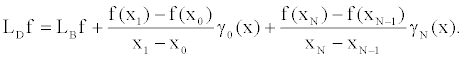(1)

In , Beatson and Powell have showed the relationship between LBf and LCf :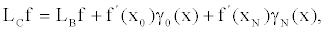(2)

where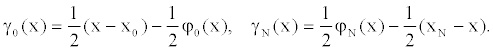For x∈[a,b] , we can easily get the two inequalities: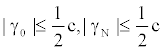(3)

Using (1), (2),(3), we can get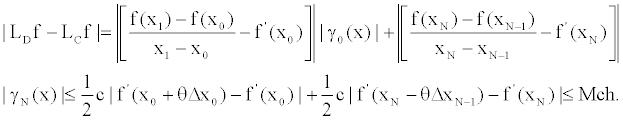Further, due to Theorem C, we can get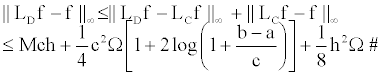Remark 1: Usually we choose c =O(h), then Theorem 1 is basically in accordance with Theorem A.

Further, for the approximated function f (x) with lower smoothness, we can get the following results:

Theorem 2: If is f(x) Lipschitz continuous in [a,b], then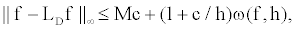where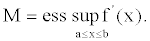Proof: Due to (3), it is obvious that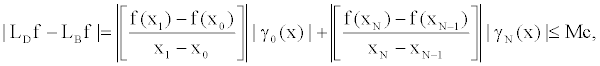Finally, using Theorem B, we have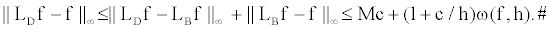Remark 2: Since f (x) is Lipschitz continuous in [a,b] and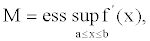we have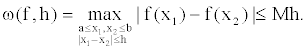Then Theorem 2 can be rewrote as:

If f (x) is Lipschitz continuous in interval [a,b] , then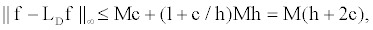whereAt last, for the general continuous approximated function f (x) , the following theorem of convergence is valid:

Theorem 3: If f (x) is continuous in [a,b] , and the interpolation knots are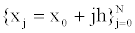(namely equally distributed), then we have the estimation: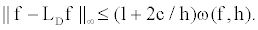Proof: Due to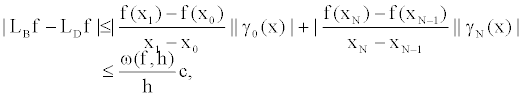using Theorem B, we have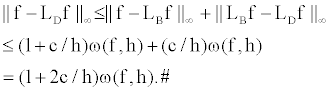Remark 3: Assuming c =O(h) in Theorem 3, we can conclude the convergence of Wu-Schaback’s quasi-interpolation operator dealing with continuous approximated functions when the interpolated knots are equally distributed.

#### Acknowledgements

Supported by the National Natural Science Foundation of China (No.11271041, No.61170005) and the Natural Science Foundation of Jilin Province (No.20130101062JC).

#### References

Select your language of interest to view the total content in your interested language

### Article Usage

• Total views: 12184
• [From(publication date):
October-2013 - Sep 19, 2019]
• Breakdown by view type
• HTML page views : 8367

## Post your commentCan't read the image? click here to refresh
###### Peer Reviewed Journals

Make the best use of Scientific Research and information from our 700 + peer reviewed, Open Access Journals

International Conferences 2019-20

Meet Inspiring Speakers and Experts at our 3000+ Global Annual Meetings

Top